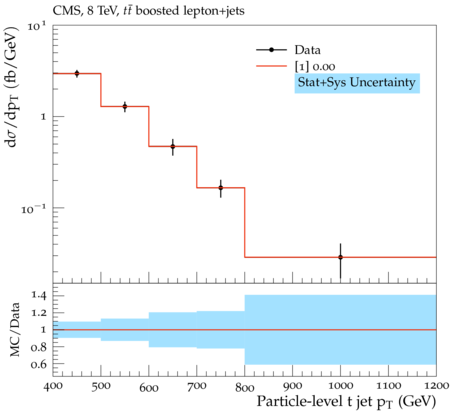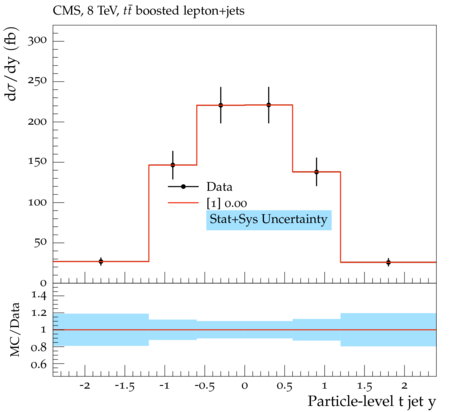### CMS_2016_I1454211

Back to index

The cross section for pair production of top quarks with high transverse momenta ($p_{\rm T} > 400$ GeV) is measured in 19.7 fb$^{-1}$ of $\mathrm{pp}$ collisions, collected with the CMS detector at $\sqrt{s} = 8$ TeV. The measurement is performed for lepton+jets events, where one top quark decays according to $t \rightarrow Wb \rightarrow \ell \nu b$, with $\ell$ denoting an electron or muon, and the second top quark decays to an hadronic final state and is reconstructed as a single, large-radius jet and identified as a top quark candidate using jet substructure techniques. Integrated cross sections, as well as differential cross sections as a function of the top quark $p_{\rm T}$ and rapidity, are measured both at particle level within a fiducial region resembling the detector-level selections and at parton level. RIVET: This analysis is to be run on ${\rm t\bar{t}}$ Monte Carlo. It utilizes the PartonicTops projection, which assumes top quarks in the event record. The analysis has been validated with Powheg+Pythia6. The parton-level phase space is defined by requiring two PartonicTops. Exactly one PartonicTop must decay directly to a muon or electron (no intermediate tau), and exactly one PartonicTop decays hadronically. For for $t\bar{t}$ Monte Carlo, this is equivalent to requiring the event to be semileptonic at parton level. The parton-level top quark is defined as the hadronically decaying top. The parton-level top quark is required to have $p_{T} > 400$ GeV. The particle-level phase space is defined using the following object definitions: - Lepton: A dressed electron or muon, meaning the lepton has been clustered with all photons within a cone of $R=0.1$. The DressedLepton projection is used to construct the dressed lepton. The lepton is required to have $p_{T} > 45$ GeV and $|\eta| < 2.1$. - B Jet Candidate: Gen AK5 jets are formed by clustering the final state particles in the event using the anti-$k_{T}$ algorithm with distance parameter $R=0.5$. Neutrinos are excluded from the clustering, as are any particles included in the dressed lepton. The gen AK5 jet is required to have $p_{T} > 30$ GeV and $|\eta| < 2.4$. Gen AK5 jets in the same hemisphere as the lepton ($\Delta {\rm R(e/\mu, jet)} < \pi/2$) are defined as b-jet candidates. - Top Jet Candidate: Gen CA8 jets are formed by clustering the final state particles in the event using the Cambridge-Aachen algorithm with distance parameter $R=0.8$. Neutrinos are excluded from the clustering, as are any particles included in the dressed lepton. The gen CA8 jet is required to have $p_{T} > 30$ GeV and $|\eta| < 2.4$. Gen CA8 jets which have $p_{T} > 400$ GeV, 140 GeV $<$ mass $<$ 250 GeV, and are in the opposite hemisphere from the lepton ($\Delta{\rm R(e/\mu, jet)} > \pi/2$) are defined as top jet candidates. The particle-level phase space is defined by requiring $\geq 1$ b jet candidate, $\geq 1$ top jet candidate, and exactly one lepton. This is in addition to the parton-level semileptonic requirement. The highest-$p_{T}$ top jet candidate is defined as the particle-level t jet.

CMS_2016_I1454211_d10-x01-y01:CMS_2016_I1454211_d12-x01-y01:Generated at Sunday, 28. January 2018 12:08PM